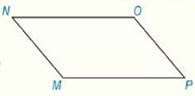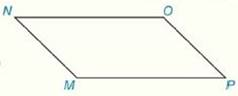Chapter 4.CR, Problem 17CR### Elementary Geometry for College St...

6th Edition
Daniel C. Alexander + 1 other
ISBN: 9781285195698

#### Solutions

Chapter
Section### Elementary Geometry for College St...

6th Edition
Daniel C. Alexander + 1 other
ISBN: 9781285195698
Textbook Problem
1 views

# Review Exercises Using the information from Review Exercise 16, determine which diagonal ( M O - or P N - ) would be longer.Exercise 16: Given: ▱ M N O P m ∠ M = 4 x m ∠ O = 2 x + 50 Find:   m ∠ M   and   m ∠ PTo determine

To Find:

The longest diagonal of the parallelogram MNOP.

Explanation

Properties Used:

1. Two consecutive angles of a parallelogram are supplementary.

2. The opposite angles of a parallelogram are congruent.

3. In a parallelogram with unequal pairs of consecutive angles, the longer diagonal lies opposite the obtuse angle.

Calculation:

It is given that MNOP is a parallelogram with mM=4x and mO=2x+50.

In the parallelogram MNOP, M and O are opposite angles.

By the property ‘opposite angles of a parallelogram are congruent’, mM=mO.

4x=2x+50

2x=50

x=25°

Substituting the value of x in measure of angle M,

mM=4x=425=100°

In the parallelogram MNOP, mM and mP are consecutive angles

### Still sussing out bartleby?

Check out a sample textbook solution.

See a sample solution

#### The Solution to Your Study Problems

Bartleby provides explanations to thousands of textbook problems written by our experts, many with advanced degrees!

Get Started

#### 39.

Mathematical Applications for the Management, Life, and Social Sciences

#### True or False: f(x) = x2 is decreasing for 10 x 1.

Study Guide for Stewart's Single Variable Calculus: Early Transcendentals, 8th

#### In Exercises 3538, find the transpose of each matrix. 

Finite Mathematics for the Managerial, Life, and Social Sciences

#### True or False: is a convergent series.

Study Guide for Stewart's Multivariable Calculus, 8th

#### Why is it necessary to have a stable pattern within a phase?

Research Methods for the Behavioral Sciences (MindTap Course List)